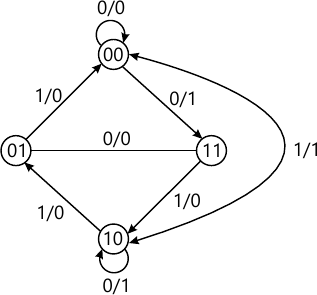Total marks: --
Total time: --
INSTRUCTIONS
(1) Assume appropriate data and state your reasons
(2) Marks are given to the right of every question
(3) Draw neat diagrams wherever necessary

1 (a) Define combinational logic. Two motors M2 and M1 are controlled by three sensors S1, S2 and S3. One motor M2 is to run any time when all three sensors are on. The other motor (M1) is to run whenever sensors S2 or S1 but not both are on and S3 is off. For all sensors combinations where M1 is on, M2 is to be off, except when all sensors are off and then both motors remain off. Construct the truth table and write the Boolean output equation.
5 M
1 (b) The following Boolean function into their proper canonical form in decimal notation.
i) M=p(q'+s)
ii) N=(w'+x)(y+z)
7 M
1 (c) Reduce the following Boolean function using K-map and realize the simplified expression using NAND gates.
T=f(a, b, c, d) = ∑m(1, 3, 4, 5, 13, 15)+ ∑d(8, 9, 10, 11).
8 M

2 (a) Simplify the following function using Quine-McClusky method and realize the simplified using NOR gates.
p=f (w,x,y,z) = ∑m(7, 9, 12, 13, 14, 15) + ∑d(4, 11)
12 M
2 (b) Simplify f(a, b, c, d) = ∑m(0, 4, 5, 6, 13, 14, 15) + ∑d(2,7,8,9) using MEV technique using basic gates.
8 M

3 (a) Design a combinational circuit to find the 9' complement of a single digit BCD number Realize the circuit using suitable logic gates.
8 M
3 (b) Draw the logic diagram for 2 to 4 line decoder with an active low encoder enable and active high data output. Construct a truth table and describe the circuit function with logic symbol (74139IC's) for the decoder.
6 M
3 (c) Design a 4 to 16 line decoder using 2 to 4 line decoder which has the active low outputs and active low enable input. Explain its operation.
6 M

4 (a) Design a binary full adder using only two input NAND gates. Write a truth table.
6 M
4 (b) Implement the following Boolean function using 4:1 multiplexer (MUX)
Y=f(A, B, C, D) = ∑m (0, 1, 2, 4, 6, 9, 12, 14)
6 M
4 (c) Define magnitude comparator. Design a two-bit binary comparator and implement with suitable logic gates.
8 M

5 (a) Discuss the difference between a flip flop and latch. Explain the operation of gated SR latch with a logic diagram, truth table and logic symbol.
6 M
5 (b) Explain the working of Master Slave JK flip flops with functional table and timing diagram. Show how race around condition is overcome.
8 M
5 (c) Obtain the characteristics equation of JK and SR flip flops.
6 M

6 (a) Describe the block diagram of a MOD-7 twisted ring counter and explain its operation with the count sequence table and decoding logic used to identify the various states.
8 M
6 (b) Design a mod-6 synchronous counter using clocked JK flipflops, the count sequence being 0, 2, 3, 6, 5, 1, 0, 2, .....
12 M

7 (a) With a suitable block diagram, explain the Mealy and Moore model, in a sequential circuit analysis.
10 M
7 (b) Explain 4-bit universal shift Register using 4:1 MUX with help of logic diagram. Write a mode control table.
10 M

8 (a) Describe the following terms with respect to sequential machines:
i) State ii) Present states iii) Next states.
6 M
8 (b) A sequential circuit has one input one output. The state diagram is shown in Fig. Q8(b). Design a sequential circuit with T flip flops.14 M

More question papers from Logic Design